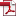Joint Time Delay and Angle Estimation Using the Matrix Pencil Method Based on Information Reconstruction Vector
• KSII Transactions on Internet and Information Systems
Monthly Online Journal (eISSN: 1976-7277)

# Joint Time Delay and Angle Estimation Using the Matrix Pencil Method Based on Information Reconstruction Vector

Vol. 12, No. 12, December 30, 201810.3837/tiis.2018.12.014 , Download Paper (Free):#### Abstract

A single snapshot data can only provide limited amount of information so that the rank of covariance matrix is not full, which is not adopted to complete the parameter estimation directly using the traditional super-resolution method. Aiming at solving the problem, a joint time delay and angle estimation using matrix pencil method based on information reconstruction vector for orthogonal frequency division multiplexing (OFDM) signal is proposed. Firstly, according to the channel frequency response vector of each array element, the algorithm reconstructs the vector data with delay and angle parameter information from both frequency and space dimensions. Then the enhanced data matrix for the extended array element is constructed, and the parameter vector of time delay and angle is estimated by the two-dimensional matrix pencil (2D MP) algorithm. Finally, the joint estimation of two-dimensional parameters is accomplished by the parameter pairing. The algorithm does not need a pseudo-spectral peak search, and the location of the target can be determined only by a single receiver, which can reduce the overhead of the positioning system. The theoretical analysis and simulation results show that the estimation accuracy of the proposed method in a single snapshot and low signal-to-noise ratio environment is much higher than that of Root Multiple Signal Classification algorithm (Root-MUSIC), and this method also achieves the higher estimation performance and efficiency with lower complexity cost compared to the one-dimensional matrix pencil algorithm.